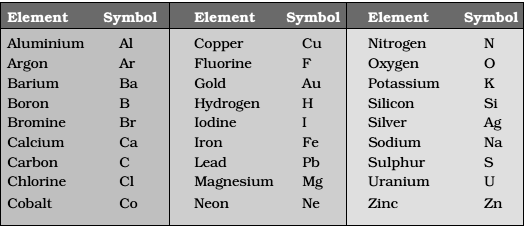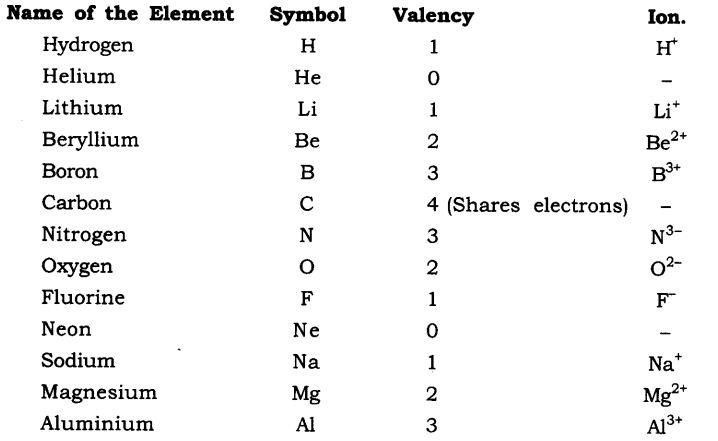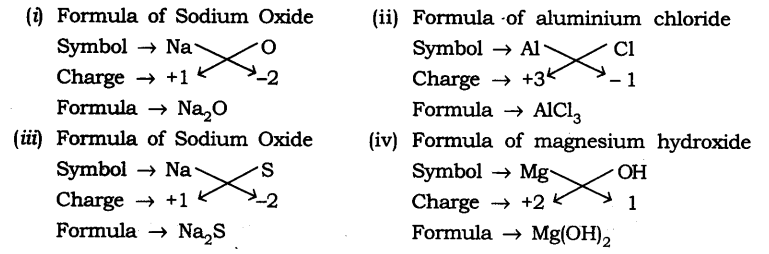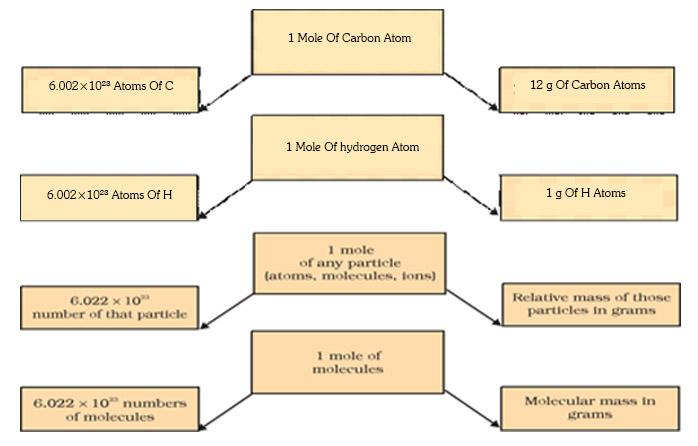×#### Thank you for registering.

One of our academic counsellors will contact you within 1 working day.

Click to Chat

1800-1023-196

+91-120-4616500

CART 0

• 0

MY CART (5)

Use Coupon: CART20 and get 20% off on all online Study Material

ITEM
DETAILS
MRP
DISCOUNT
FINAL PRICE
Total Price: Rs.

There are no items in this cart.
Continue Shopping• Complete JEE Main/Advanced Course and Test Series
• OFFERED PRICE: Rs. 15,900
• View Details

```Revision Notes on Atoms and Molecules

The invisible and unknown form of matter

The idea of divisibility by Indian philosophers

Maharishi Kanad – He postulated that if we keep on dividing the matter (called as ‘padarth’) we will get smaller and smaller particles.  And soon we will achieve the smallest of particles (called as ‘parmanu’) which may not divide further.

Pakudha Katyayama – He postulated that there are various forms of matter because the particles of matter exist together in combinations.

The idea of divisibility by Greek philosophers

Democritus and Leucippus – They suggested that when we keep on dividing the matter there comes a time when no more division of particles can take place. Such particles are called atoms which means being invisible.

But all these ideas were not backed up by many experimental pieces of evidence until Antoine L. Lavoisier provided two laws of chemical combination.

Laws of Chemical Combination

1. Law of conservation of mass – mass can neither be created nor destroyed in a chemical reaction

2. Law of constant proportion/Law of definite proportion – the elements are always present in definite proportions by mass in a chemical substance

For example, Hydrogen and oxygen are present in water in a ratio of 1:8. So if we decompose 9g of water we will obtain 1g of hydrogen and 8g of oxygen.

The Atomic Theory

John Dalton proposed an atomic theory which acted as an explanation of the above two laws. As per the theory, all matter whether it is an element, a compound or a mixture consists of tiny invisible particles called ‘atoms’.

The postulates of the atomic theory by John Dalton

1. The matter is made up of tiny particles called atoms that cannot be divided.

2. Atoms are never formed or destroyed during a chemical reaction.

3. Atoms of an element exhibit same nature. They have the same size, mass, and character.

4. Atoms of different elements exhibit variant nature. They do not have same characteristics.

5. Atoms form compounds by combining in a ratio of whole numbers.

6. A compound contains a constant number and kinds of atoms

Atoms

We can call atoms as the building blocks of matter. Just like bricks are the building blocks of a building.

What is the size of an atom?

Atoms are extremely small. Their size is measured in nanometers where 1nm = 1/109 m.

Atomic radius is measured in nanometers
1/109 = 1nm
1m  = 109 nm

Relative Sizes

Example

10-10
Atom of hydrogen

10-9
Molecule of water

10-8
Molecule of haemoglobin

10-4
Grain of Sand

10-2
Ant

10-1
Watermelon

Symbols for Atoms

Here are some examples of the symbols that are used to represent different atomsThe symbols for representing an atom are generated from the first two letters of the element’s name. The first letter is always in uppercase (capital letter) while the second letter is written in lowercase. Here are some examples –The Atomic Mass

The Dalton’s Atomic Theory suggested that each element has a distinguishing atomic mass. With this theory, the law of constant proportions could be explained easily.

But it is indeed difficult to evaluate the mass of an atom since the size of an atom is relatively small.

Therefore scientists started evaluating the mass of an atom by comparing it with the mass of a standard atom.

Earlier 1/16 of the mass of an oxygen atom was used as a standard for calculating the mass of other elements. Now, carbon - 12 is considered a standard atom for calculating the mass.

Its atomic mass is 12u (12 atomic mass units). Thus we can say that one atomic mass unit is the mass of 1/12 the mass of a carbon-12 atom. Here is a list of atomic masses of a few elements.

Element
Atomic Mass

Hydrogen
1 µ

Carbon
12 µ

Nitrogen
14 µ

Oxygen
16 µ

Sodium
23 µ

Magnesium
24 µ

Sulphur
32 µ

Chlorine
35.5 µ

Calcium
40 µ

Can atoms exist independently?

Atoms cannot survive independently. So, atoms join together and form molecules or ions.

Molecule

A molecule is a collection of various atoms that combine chemically with each other.

These atoms are bound together by certain forces of attraction.

Atoms of the same elements or different elements can bind together to form molecules.

Therefore, a molecule is the smallest particle of a substance that can exist independently and shows all the properties of that substance.

Molecules of Elements

The molecules of an element are formed by combinations of similar types of atoms. For example, Helium (He) is made up of only one atom while oxygen is made up of two atoms.

Atomicity – the number of atoms in a molecule of an element is called its atomicity. For example, helium is monoatomic and oxygen is diatomic.

Monoatomic – when an element comprises of a single atom. Example – all metals

Diatomic – when an element comprises of two atoms. Example – all gases

Triatomic – when an element comprises of three atoms

Tetra-atomic – when an element comprises of four atoms

Poly-atomic – when an element comprises of more than two atoms

Here a few examples of atomicity of elements –

Atomicity of some Elements

Name
Atomicity
Formula

Argon
Monoatomic
Ar

Helium
Monoatomic
He

Oxygen
Diatomic
O2

Hydrogen
Diatomic
H2

Nitrogen
Diatomic
N2

Chlorine
Diatomic
Cl2

Phosphorous
Tetra – atomic
P4

Sulphur
Poly – atomic
S8

Molecules of Compounds

Molecules of compounds constitute atoms of different elements that combine together in a fixed proportion. For example, water comprises of two atoms of hydrogen and one atom of oxygen.Ions

Compounds contain metals as well as non-metals. These elements include charged species which are known as ions.

Thus, ion is a particle that has a positive or negative charge.

Anion – negatively charged ion

Cation – positively charged ion

There can be a single charged atom in an ion or there may be a group of charged atoms in an ion that have a net charge on the compound.

When a group of atoms carries a charge in a compound it is called as a polyatomic ion.

Chemical Formula

We use a chemical formula to represent the composition of a compound in the form of symbols. To write a chemical formula you must know two things –

1. Symbols of elements

2. Valency

Valency – it is also known as the combining capacity of an element. In other words, valency explains how atoms of one element will mix with atoms of another element. For example, the hydrogen ion is represented as H+ which means that its valency is 1. Similarly, the oxygen ion is represented as O2- which means that its valency is 2. Here is a list of valancies of various elements.Rules of writing a Chemical Formula

Valencies of on the ions must balance.

In a case where both metal and non-metal substances are present in a compound, the name of the metal is always written first in the chemical formula. For example, Sodium Chloride is written as NaCl

In case of polyatomic ions, the ion is written in brackets before writing the number of ions associated to it. In case of a single ion, there is no need to mention the ion in brackets

Writing the Formulae of Simple Compounds

Binary compounds – compounds that consist of two different elements

How to write a Formula of a Compound

Write the symbols of the corresponding elements of the compound as explained above

Write the valencies of the elements of the compound

Crossover the valencies of the elements

Here are a few examples of writing the chemical formulaMolecular Mass and the Mole Concept

Molecular Mass – summation of all the atomic masses in a molecule

Molecular mass is expressed in atomic mass units (amu).

For example, the molecular mass of HNO3 can be calculated as:

Atomic mass of H =1u

Atomic mass of N =14u

Atomic mass of O =16u

Molecular mass of HNO3 = 1 + 14 + (16*3) = 63u

Formula Unit Mass

The sum of atomic masses of all atoms in a formula unit of a compound is called as its formula unit mass.  The formula unit mass is used in case of substances that constitute ions. For example, formula unit mass of Sodium Chloride (NaCl) can be calculated as:    (1*23) + (1*35.5) = 58.5uMole Concept

How do we interpret a chemical equation?

2C + O2 = 2CO2

We say that two molecules of carbon combine with one molecule of oxygen to form two molecules of carbon dioxide.

We can also say that 24u of Carbon molecules combine with 32u of oxygen molecules to form 56u of carbon dioxide molecules.

Therefore, we can characterize the quantity of a substance by its mass or by its number of molecules.

A chemical equation directly indicates the number of molecules participating in the reaction. Thus, it is convenient for us to refer to the number of substances in a chemical reaction as numbers of molecules or atoms.

Mole

Mole is a numerical quantity that has a mass equal to the atomic or molecular mass of species (atoms, molecules, ions or particles).

1 mole of any substance = 6.022 X 1023 number of particles (atoms, ions or molecules)

This is called the Avogadro number or Avogadro Constant which is represented as N0

The mass of 1 mole of a substance is the same as that its atomic mass or molecular mass expressed in grams.

Gram atomic mass of a substance – the atomic mass of a substance when expressed in grams is known as its gram atomic mass.

Gram molecular mass of a substance – the molecular mass of a substance when expressed in grams is known as its gram molecular mass.

For example, the atomic mass of Sulphur is 32u. Gram atomic mass of Sulphur is 32g.

Also, 32u of Sulphur has 1 atom of Sulphur. 32g of Sulphur has 1 mole atoms, that is, 6.022 X 1023 atoms of Sulphur.

Similarly, we can say that the gram molecular mass of Carbon Dioxide is 56g.

But we know that in the case of chemical equation mole is the measuring unit.

Therefore, 1 mole = 6.022 × 1023 number = Relative mass in grams

Wilhelm Ostwald introduced the word ‘mole’ which actually means a heap or a pile. Therefore, we consider a substance as a heap of atoms or molecules.

Consider these formulae –A quick review of how mole, Avogadro number and Mass are related to each other –```### Course Features

• 728 Video Lectures
• Revision Notes
• Previous Year Papers
• Mind Map
• Study Planner
• NCERT Solutions
• Discussion Forum
• Test paper with Video Solution